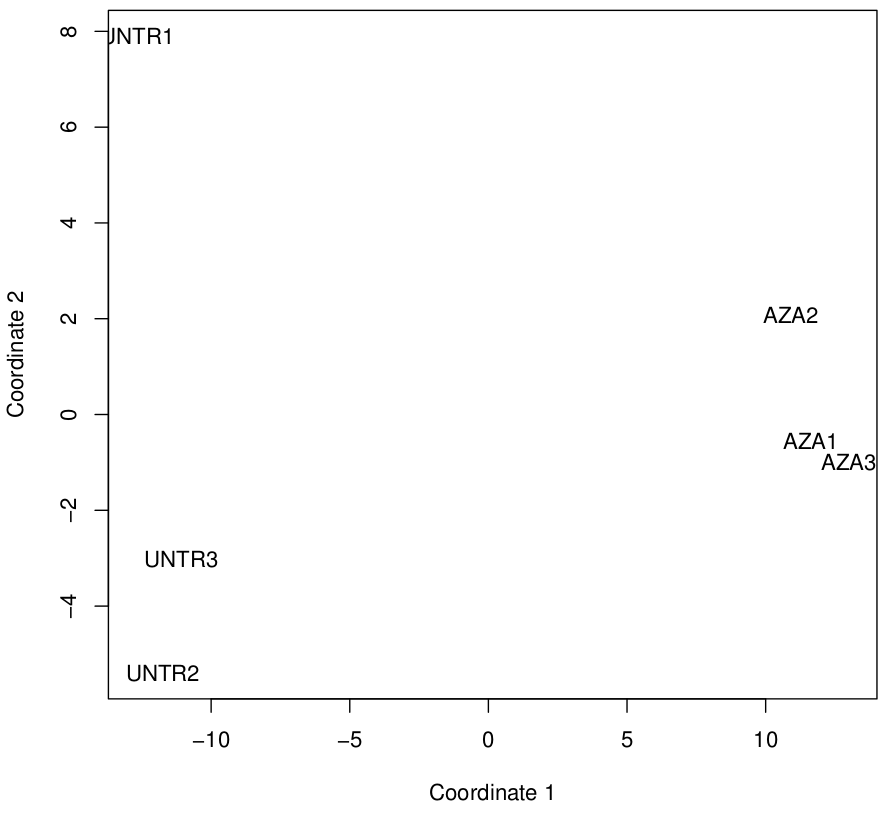### Data analysis step 7: Fast MDS plot

We will continue our series in the analysis of our azacitidine treated AML3 cell RNA-seq gene expression data set by generating a multidimensional scaling plot. This is a potentially useful way of showing variability in datasets, especially when the number of samples is large. Trawling the blogs, I found a really quick and easy way to do this in R (thanks Michael Dondrup@BioStars) that can be used to analyse the count matrix.

``` x<-scale(read.table("CountMatrix.xls", row.names=1, header=TRUE))```
`pdf("MDSplot.pdf") `
`plot(cmdscale(dist(t(x))), xlab="Coordinate 1", ylab="Coordinate 2", type = "n") ; text(cmdscale(dist(t(x))), labels=colnames(x), ) `
`dev.off()`Multidimensional scaling (MDS) plot for public gene expression data (GSE55123).
The closer the labels are together, the more similar the samples are. So it is good to see that the untreated samples are clearly separated from the azacitidine treated samples. UNTR1 is a bit far away from the other two replicates as suggested by the heatmap in the previous post.